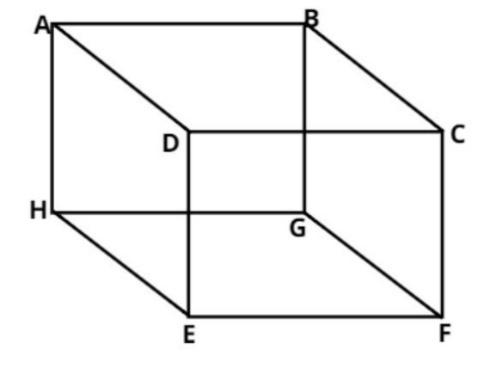QUESTION

# Find the lateral surface area (L.S.A) and the total surface area (T.S.A) and volume of a cube of side 5cm.

Hint: In this question use the direct formula for lateral surface area of cube which is$4{a^2}$, the total surface area of cube which is $6{a^2}$ and the volume of cube which is ${a^3}{\text{ c}}{{\text{m}}^3}$.The pictorial representation of the cube is shown above.
As we know that in a cube all the sides are equal.
Let us suppose that the side is a cm.
And it is given that the side of the cube is 5 cm.
$\Rightarrow a = 5$ cm.
Now as we know that the cube has 6 faces and all represents a square.
And we all know that the area of the square is (side) 2.
So the total surface area (T.S.A) of the cube is$= 6{a^2}$.
$\Rightarrow T.S.A = 6{\left( 5 \right)^2} = 150{\text{ c}}{{\text{m}}^2}$
Now as we know that in the lateral surface area (L.S.A) of a cube top and bottom faces area is neglected (i.e. in L.S.A of a cube there are only 4 faces).
So the lateral surface area (L.S.A) of the cube is$= 4{a^2}$.
$\Rightarrow L.S.A = 4{\left( 5 \right)^2} = 100{\text{ c}}{{\text{m}}^2}$
Now as we know that the volume (V) of the cube is side cube.
So the volume (V) of the cube is$= {a^3}{\text{ c}}{{\text{m}}^3}$.
$\Rightarrow V = {5^3} = 125{\text{ c}}{{\text{m}}^3}$
So the L.S.A, T.S.A and the volume of the cube are 100 square centimeter, 150 square centimeter and 125 cubic centimeter.
So this is the required answer.

Note: The main confusion that is still here is how the lateral surface area is different from total surface area and why it’s formulas are different?
Lateral surface area is the area of all sides excluding the bases, so in a cube there are 6 sides and if bases are excluded which are two in number one on top and one on bottom only 4 sides are left thus it’s formula is $4{a^2}$, however total surface area is the area of all the sides including the bases so there are in total 6 sides thus the formula is $6{a^2}$.